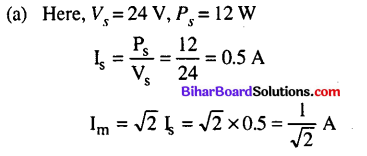# Bihar Board 12th Physics Objective Answers Chapter 7 Alternating Current

Bihar Board 12th Physics Objective Questions and Answers

## Bihar Board 12th Physics Objective Answers Chapter 7 Alternating Current

Question 1.
Alternating voltage (V) is represented by the equation
(a) V(t)=Vmewt
(b) V(t) = Vm sinωt
(c) V(t) = Vm cosωt
(d) V(t) = Vm tanωt
where Vm is the peak voltage
(b) V(t) = Vm sinωt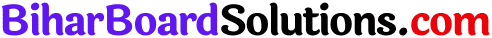Question 2.
A 100 Ω resistor is connected to a 220 V, 50 Hz ac supply. The rms value of current in the circuit is
(a) 1.56 A
(b) 1.56 mA
(c) 2.2 A
(d) 2.2 mA
(c) 2.2 A

Question 3.
The peak voltage of an ac supply is 440 V, then its rms voltage is
(a) 31.11 V
(b) 311.1 V
(c) 41.11V
(d) 411.1V
(b) 311.1 V

Question 4.
The rms value of current in an ac circuit is 25 A, then peak current is
(a) 35.36 mA
(b) 35.36 A
(c) 3.536 A
(d) 49.38 A
(b) 35.36 AQuestion 5.
A light bulb is rated at 100 W for a 220 V ac supply. The resistance of the bulb is
(a) 284 Ω
(b) 384 Ω
(c) 484 Ω
(d) 584 Ω
(c) 484 Ω
Solution:
(c) Here, P = 100 W, = 220 V
Resistance of the bulb is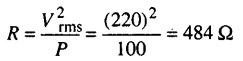Question 6.
An ac source is of $$\frac{200}{\sqrt{2}} \mathbf{v}$$, 50 Hz. The value of voltage
after $$\frac{1}{600}$$ from the start is
(a) 200V
(b) $$\frac{200}{\sqrt{2}} \mathbf{v}$$
(c) 100V
(d) 50V
(c) 100V
Solution: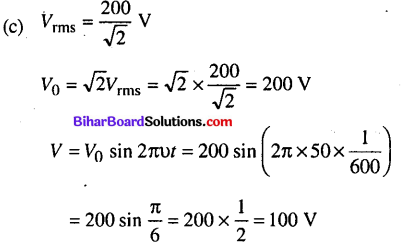Question 7.
An ac source of voltage V = Vm sincot is connected across the resistance R as shown in figure. The phase relation between current and voltage for this circuit is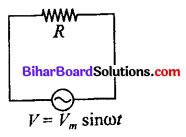(a) both are in phase
(b) both are out of phase by 90°
(c) both are out of phase by 120°
(d) both are out of phase by 180°
(a) both are in phaseQuestion 8.
In the case of an inductor
(a) voltage lags the current by $$\frac{\pi}{2}$$
(b) voltage leads the current by $$\frac{\pi}{2}$$
(c) voltage lags the current by $$\frac{\pi}{3}$$
(d) voltage leads the current by $$\frac{\pi}{4}$$
(b) voltage leads the current by $$\frac{\pi}{2}$$

Question 9.
An ideal inductor is in turn put across 220 V, 50 Hz and 220 V, 100 Hz supplies. The current flowing through it in the two cases will be
(a) equal
(b) different
(c) zero
(d) infinite
(b) differentQuestion 10.
An inductor of 30 mH is connected to a 220 V, 100 Hz ac source. The inductive reactance is
(a) 1058 Ω
(b) 12.64 Ω
(c) 18.85 Ω
(d) 22.67 Ω
(c) 18.85 Ω
Solution:
(c) Here, L = 30 mH = 30 × 10-3 H; Vrms = 220 V,
υ = 100 Hz
Inductive reactance XL = 2πυL
= 2 × 3.14 × 100 × 30 × 10-3
= 18.85 Ω

Question 11.
A 44 mH inductor is connected to 220 V, 50 Hz ac supply. The rms value of the current in the circuit is
(a) 12.8 A
(b) 13.6 A
(c) 15.9 A
(d) 19.5 A
(c) 15.9 A
Solution:
(c) Here, L = 44 mH = 44 × 10-3 H; Vrms= 220V,
υ = 50 Hz
The inductive reactance is XL = ωL
= 2πυL = 2 × 3.14 × 50 × 44 × 10-3
= 13.82 Ω
∴ $$\quad I_{\mathrm{rms}}=\frac{V_{\mathrm{rms}}}{X_{L}}=\frac{220}{13.82}=15.9 \mathrm{A}$$

Question 12.
A 5 μF capacitor is connected to a 200 V, 100 Hz ac source. The capacitive reactance is
(a) 212 Ω
(b) 312 Ω
(c) 318 Ω
(d) 412 Ω
(c) 318 ΩQuestion 13.
If a capacitor of 8 ΩF is connected to a 220 V, 100 Hz ac source and the current passing trough it is 65 mA, then the rms voltages across it is
(a) 129.4 V
(b) 12.94 V
(c) 1.294 V
(d) 15 V
(b) 12.94 V
Solution:
(b) Here, Vrms = 220 V,Irms = 65 mA = 0.065 A
C = 8 μF = 8 × 10-6 F, υ = 100 Hz
Capacitive reactance,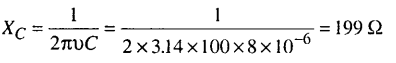Then rms voltage across the capacitor is
VCrms = 7rmsXC = 0.065 × 199= 12.94 V

Question 14.
Phase difference between voltage and current in a capacitor in an ac circuit is
(a) π
(b) π/2
(c) 0
(d) π/3
(b) π/2

Question 15.
A 30 μF capacitor is connected to a 150 V, 60 Hz ac supply. The rms value of current in the circuit is
(a) 17 A
(b) 1.7 A
(c) 1.7 mA
(d) 2.7 A
(b) 1.7 A
Solution:
(b) Here, C = 30 × 10-6 F, Vrms = 150 V, υ = 60 Hz
Capacitive reactance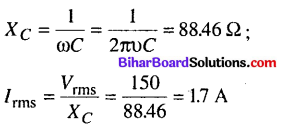Question 16.
A 60 μF capacitor is connected to a 110 V (rms), 60 Hz ac supply. The rms value of current in the circuit is
(a) 1.49 A
(b) 14.9 A
(c) 2.49 A
(d) 24.9 A
(c) 2.49 A

Question 17.
In which of the following circuits the maximum power dissipation is observed ?
(a) Pure capacitive circuit
(b) Pure inductive circuit
(c) Pure resistive circuit
(d) None of these
(c) Pure resistive circuit

Question 18.
When an ac voltage of 220 V is applied to the capacitor C, then
(a) the maximum voltage between plates is 220 V.
(b) the current is in phase with the applied voltage.
(c) the charge on the plate is not in phase with the applied voltage.
(d) power delivered to the capacitor per cycle is zero.
(b) the current is in phase with the applied voltage.

Question 19.
In the series LCR circuit shown the impedance is
(a) 200 Ω
(b) 100 Ω
(c) 300 Ω
(d) 500 Ω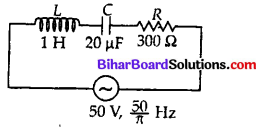(d) 500 Ω
Solution:
(d) Here, L = 1 H, C = 20 μF = 20 × 10-6F; R = 300 Ω, υ = 50/π Hz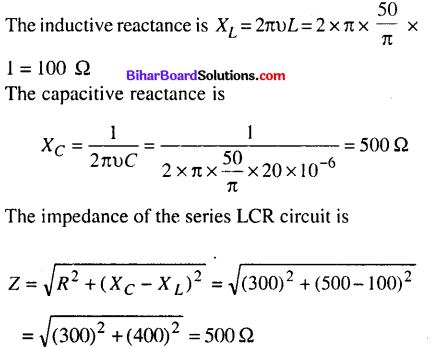Question 20.
A 100 μF capacitor in series with a 40 Ω resistor is connected to a 100 V, 60 Hz supply. The maximum current in the circuit is
(a) 2.65 A
(b) 2.75 A
(c) 2.85 A
(d) 2.95 A
(d) 2.95 A
Solution:
(d) Here, C = 100 μ.F = 100 × 10-6 F = 10-4– F,
R = 40 Ω, Vrms = 100 V,υ = 60 Hz
V0 = √2Vrms = 100√2V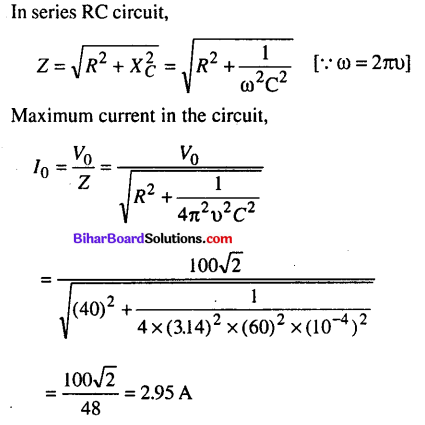Question 21.
In series LCR circuit, the phase angle between supply voltage & current is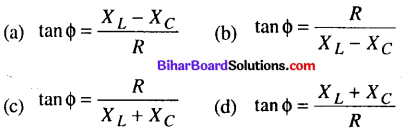$$\tan \phi=\frac{X_{L}-X_{C}}{R}$$

Question 22.
In a circuit L, C and R are connected in series with an alternating voltage source of frequency υ . The current leads the voltage by 45°. The value of C is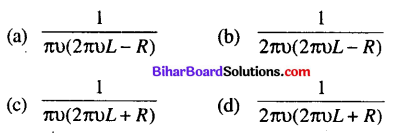(d) $$\frac{1}{2 \pi v(2 \pi v L+R)}$$

Question 23.
At resonance frequency the impedance in series LCR cirucit is
(a) maximum
(b) minimum
(c) zero
(d) infinity
(b) minimumQuestion 24.
At resonant frequency the current amplitude in series LCR circuit is
(a) maximum
(b) minimum
(c) zero
(d) infinity
(a) maximum

Question 25.
The resonant frequency of a series LCR circuit with L = 2.0 H, C = 32 μF and R = 10 Ω is
(a) 20 Hz
(b) 30 Hz
(c) 40 Hz
(d) 50 Hz
(a) 20 Hz

Question 26.
The Q factor of a series LCR circuit with L = 2 H, C=32 μF and R = 10 Ω is
(a) 15
(b) 20
(c) 25
(d) 30
(c) 25

Question 27.
A series LCR circuit has R = 5 Ω, L = 40 mH and C = 1 μF, the bandwidth of the cirucit is
(a) 10 Hz
(b) 20 Hz
(c) 30 Hz
(d) 40 Hz
(b) 20 Hz

Question 28.
In LCR-circuit if resistance increases, quality factor
(a) increases finitely
(b) decreases finitely
(c) remains constant
(d) none of these
(b) decreases finitelyQuestion 29.
A series resonant LCR circuit has a quality factor (Q- factor) = 0.4. If R = 2k Ω , C=0.1 μF, then the value of inductance is
(a) 0.1 H
(b) 0.064 H
(c) 2H
(d) 5 H
(b) 0.064 H
Solution:
(b) Quality factor $$Q=\frac{1}{R} \sqrt{\frac{L}{C}} \text { or } \frac{L}{C}=(\mathrm{QR})^{2}$$
Here, Q = 0.4, R = 2k Ω = 2 × 103 Ω;
C = 0.1 μ.F = 0.1 × 10-6
∴ L = (QR)2  C
∴ L = (0.4 × 2 × 103)2 × 0.1 × 10-6 = 0.064 H

Question 30.
An alternating supply of 220 V is applied across a circuit with resistance 22 Ω and impedance 44 Ω. The power dissipated in the cirucit is
(a) 1100 W
(b) 550 W
(c) 2200 W
(d) (2200/3) W
(b) 550 W
Solution:
(b) Here, V = 220 V, Resistance, R = 22 Ω
Impedance, Z = 44 Ω
Current in cirucit, $$I=\frac{V}{Z}=\frac{220 \mathrm{V}}{44 \Omega}=5 \mathrm{A}$$
Power dissipated in the circuit,
p = I2R = (5)2 × 22 = 550 WQuestion 31.
In a series LCR circuit, the phase difference between the voltage and the current is 45°. Then the power factor will be
(a) 0.607
(b) 0.707
(c) 0.808
(d) 1
(b) 0.707
Solution:
(b) Here, Φ = 45° In series LCR circuit,
power factor = cosΦ
cos Φ = cos 45° = $$\frac{1}{\sqrt{2}}$$ = 0.707.

Question 32.
The natural frequency (ω0) of oscillations in LC circuit is given by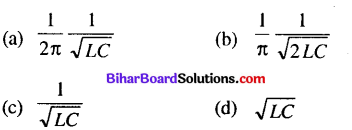(c) $$\frac{1}{\sqrt{L C}}$$

Question 33.
An LC circuit contains a 20 mH inductor and a 25 pF capacitor with an initial charge of 5 mC. The total energy stored in the circuit initially is
(a) 5 J
(b) 0.5 J
(c) 50 J
(d) 500 J
(b) 0.5 J
Solution:
(b) Here, C = 25 µF = 25 × 10-6 F,
L = 20mH = 20 × 10-3H, q0
= 5 mC = 5 × 10-3 C
∴ Total energy stored in the circuit initially is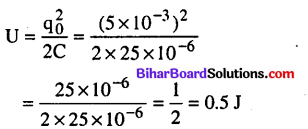Question 34.
What is the mechanical equivalent of spring constant k in LC oscillating circuit ?
(a) $$\frac{1}{L}$$
(b) $$\frac{1}{C}$$
(c) $$\frac{L}{C}$$
(d) $$\frac{1}{LC}$$
(b) $$\frac{1}{C}$$

Question 35.
A transformer works on the principle of
(a) self induction
(b) electrical inertia
(c) mutual induction
(d) magnetic effect of the electrical current
(c) mutual induction

Question 36.
Transformer is used to
(a) convert ac to dc voltage
(b) convert dc to ac voltage
(c) obtain desired dc power
(d) obtain desired ac voltage and current
(d) obtain desired ac voltage and current

Question 37.
Quantity that remains unchanged in a transformer is
(a) voltage
(b) current
(c) frequency
(d) none of these
(c) frequencyQuestion 38.
The core of a transformer is laminated to reduce
(a) flux leakage
(b) hysteresis
(c) copper loss
(d) eddy current
(d) eddy current

Question 39.
The loss of energy in the form of heat in the iron core of a transformer is
(a) iron loss
(b) copper loss
(c) mechanical loss
(d) none of these
(a) iron loss

Question 40.
A transformer &used to light 140 W, 24 V lamp from a 240 V at mains. If the main current is 0.7 A, the efficiency of the transformer is
(a) 63,0%
(b) 74%
(c) 83.3%
(d) 48%
(c) 83.3%
(c) Output power = 140 W,
Inpute power = 240 × 0.7 = 168 W
Efficiency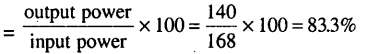Question 41.
If the rms current in a 50 Hz ac circuit is 5 A, the value of the current 1/300 seconds after its value becomes zero is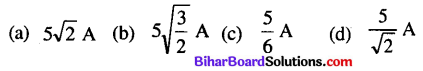(b) $$5 \sqrt{\frac{3}{2}} \mathrm{A}$$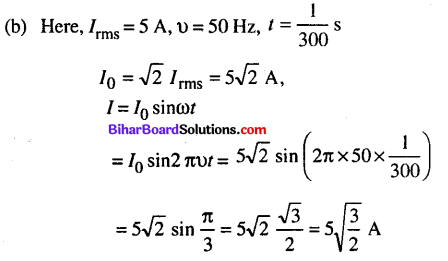Question 42.
An inductor of reactance 1Ω and a resistor of 2Ω are connected in series to the terminals of a 6 V (rms) ac source. The power dissipated in the circuit is
(a) 8 W
(b) 12 W
(c) 14.4 W
(d) 18 W
(c) 14.4 WQuestion 43.
The output of a step-down transformer is measured to be 24 V when connected to a 12 watt light bulb. The value of the peak current is
(a) $$\frac{1}{\sqrt{2}} \mathrm{A}$$
(b) √2A
(c) 2 A
(d) 2√2 A
(a) $$\frac{1}{\sqrt{2}} \mathrm{A}$$